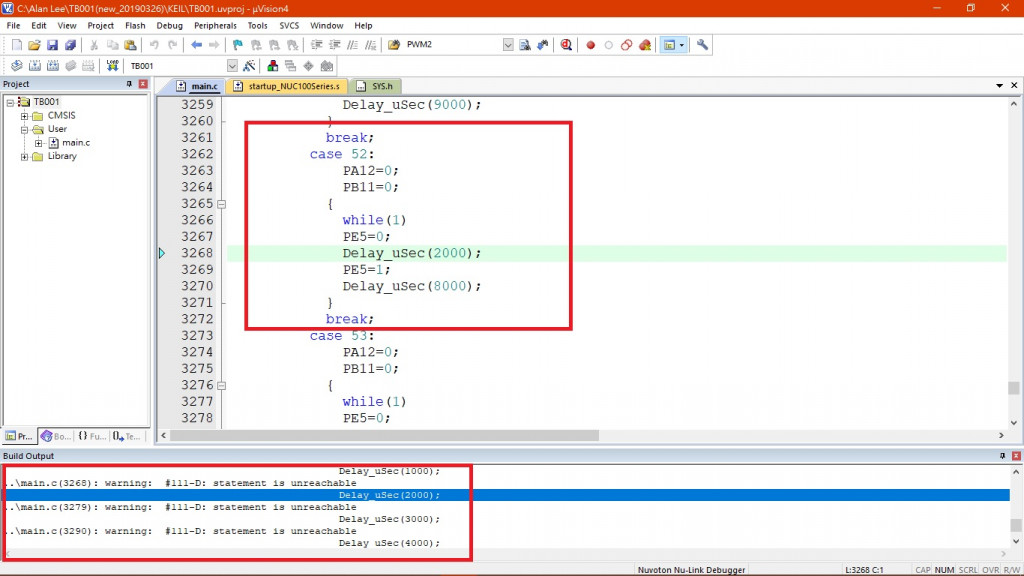#0

## 想請教一個問題### 2 個回答

1

warning訊息是`「statement is unreachable」`

``````3266 while(1)   //這是永不停止的無窮迴圈
3267    PE5=0;  //而迴圈裡只會執行這列
``````

``````3262 case 52: {
3263    PA12 = 0;
3264    PB11 = 0;
//3265    {                 //刪掉
//3266    while(1)          //刪掉
3267    PE5 = 0;
3268    Delay_uSec(2000);
3269    PE5 = 1;
3270    Delay_uSec(8000);
3271    }
3272    break;
``````

while(1)是「無窮迴圈」，是「永遠執行不會離開」的情境

INT中斷控制???

1

``````int count1=0,count2=10000;

while(1)
{
PE5=0;
Delay_uSec(count1);
PE5=1;
Delay_uSec(count2);

if(count1>=10000)
{
count1=0;
count2=10000;
}
else
{
count1+=1000;
count2-=1000;
}
}
``````

count數字再自己調看看